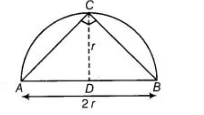# Area of the largest triangle that can

Question:

Area of the largest triangle that can be inscribed in a semi-circle of radius r units is

(a) $r^{2}$ squnits

(b) $\frac{1}{2} r^{2}$ sq units

(c) $2 r^{2}$ sq units

(d) $\sqrt{2} r^{2}$ sq units

Solution:

(a) Take a point $\mathrm{C}$ on the circumference of the semi-circle and join it by the end points of diameter $\mathrm{A}$ and $\mathrm{B}$.

$\therefore \quad \angle C=90^{\circ}$ [by property of circle]

[angle in a semi-circle are right angle]

So, $\triangle A B C$ is right angled triangle.$\therefore$ Area of largest $\triangle A B C=\frac{1}{2} \times A B \times C D$

$=\frac{1}{2} \times 2 r \times r$

$=r^{2}$ sq units